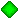#Japanese version       Home Page       Updated on May 10, 1999

# Distance

It calculates the distance and position angle between two coordinates.

##Execution

When using Windows95, open DOS Prompt and type:
```    java misao.app.Distance
```
If JRE(Java Runtime Environment) is installed, the directory where the package is unpacked must be set by an option -cp (the example is in case the package is unpacked at C:\).
```    java -cp C:\ misao.app.Distance
```
Then the following messages are output, so input the first pair of R.A. and Decl.
```Calculate angular distance between two positions.
Input positions:
ex. R.A.= 12h 4m31.2s  Decl.= - 0o15'
acceptable style: 12 4 31.2 -0 15 0
Position 1:
```
Continuously, input the second pair of R.A. and Decl. following the message:
```Position 2:
```
Then the distance of the two coordinates are output in arcsec, arcmin and deg. And the position angle from the first position to the second one is also output.

PIXY System Home Page

Copyright(C) MISAO Project (comet@aerith.net). All rights reserved.